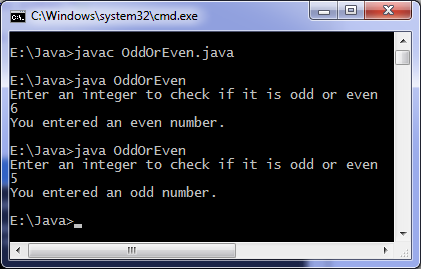# To find odd or even

Levels of difficulty: / perform operation:

This java program finds if a number is odd or even. If the number is divisible by 2 then it will be even, otherwise it is odd. We use modulus operator to find remainder in our program.

## Java source code: Example 1

```import java.util.Scanner;

class OddOrEven
{
public static void main(String args[])
{
int x;
System.out.println("Enter an integer to check if it is odd or even ");
Scanner in = new Scanner(System.in);
x = in.nextInt();

if ( x % 2 == 0 )
System.out.println("You entered an even number.");
else
System.out.println("You entered an odd number.");
}
}
```

## Output:1## Java source code: Example 2

```import java.util.Scanner;

class EvenOdd
{
public static void main(String args[])
{
int c;
System.out.println("Input an integer");
Scanner in = new Scanner(System.in);
c = in.nextInt();

if ( (c/2)*2 == c )
System.out.println("Even");
else
System.out.println("Odd");
}
}
```